# RS Aggarwal Solutions for Class 6 Chapter 4 Integers Exercise 4B

RS Aggarwal Solutions for Class 6, Chapter 4, Integers Exercise 4B are provided here. These solutions are prepared by expert tutors in Mathematics with detailed explanations in simple language. This helps the students gain a strong foundation in Maths, thus helping them to score good marks. Students can refer RS Aggarwal solutions to practice exercise-wise problems to gain a better understanding of concepts.

## Download PDF of RS Aggarwal Solutions for Class 6 Chapter 4 Integers Exercise 4B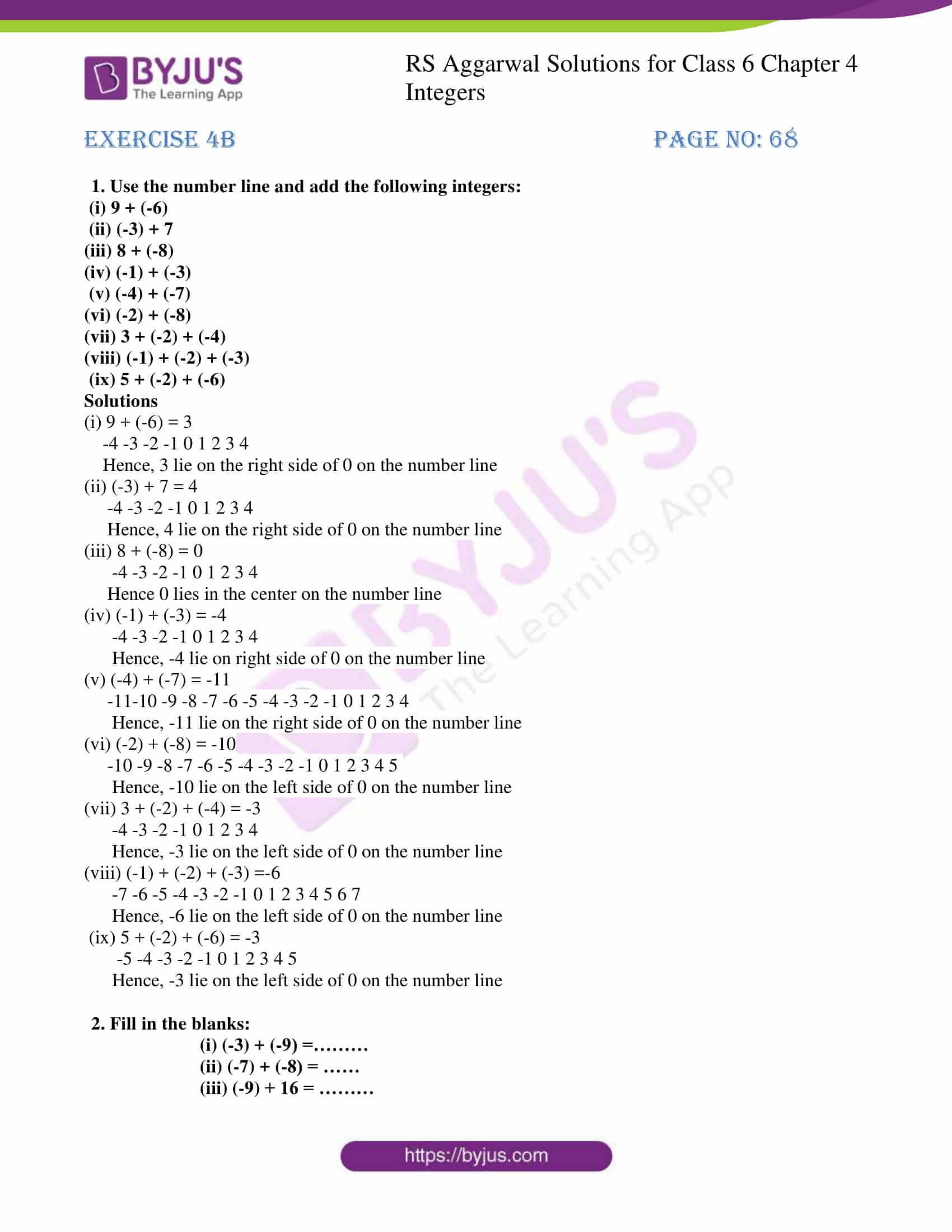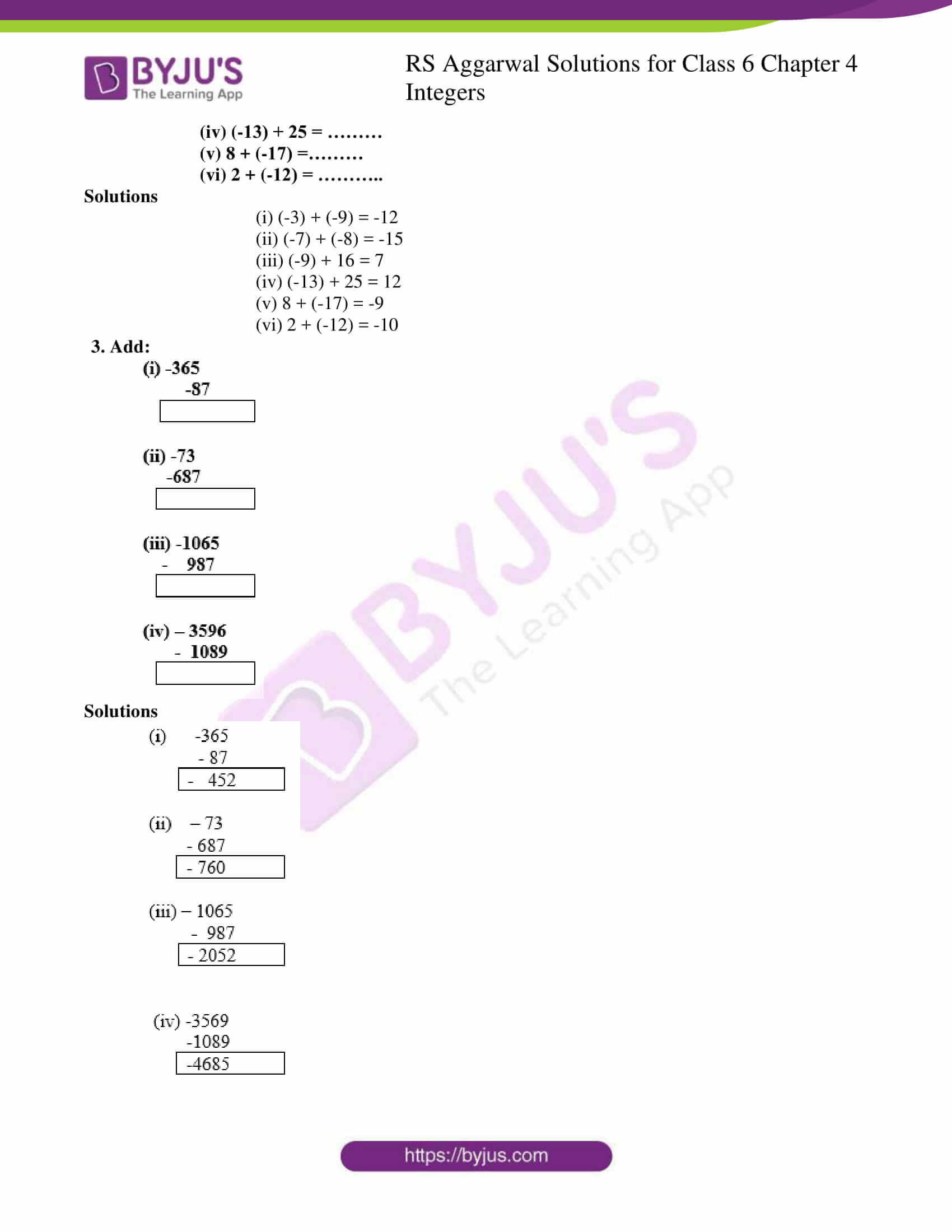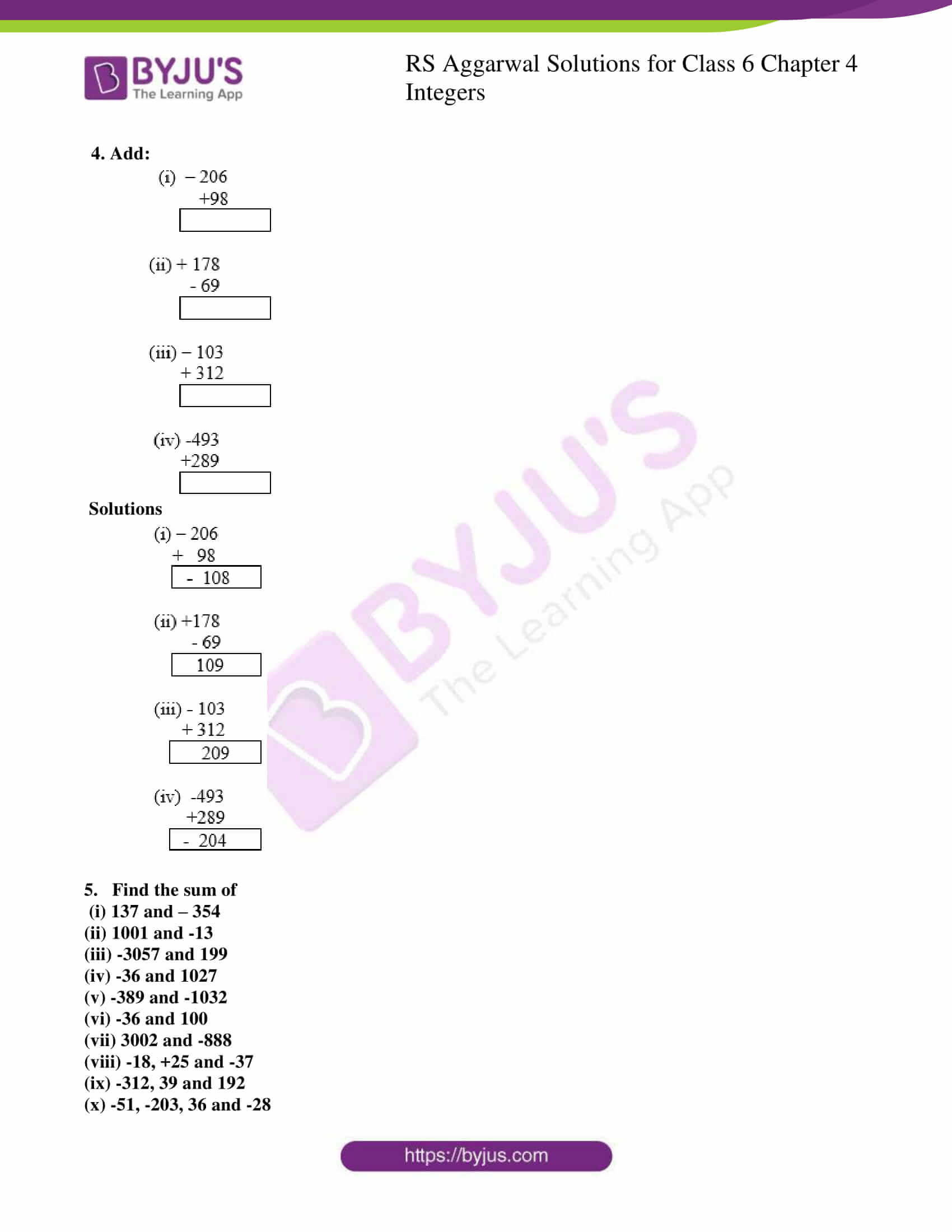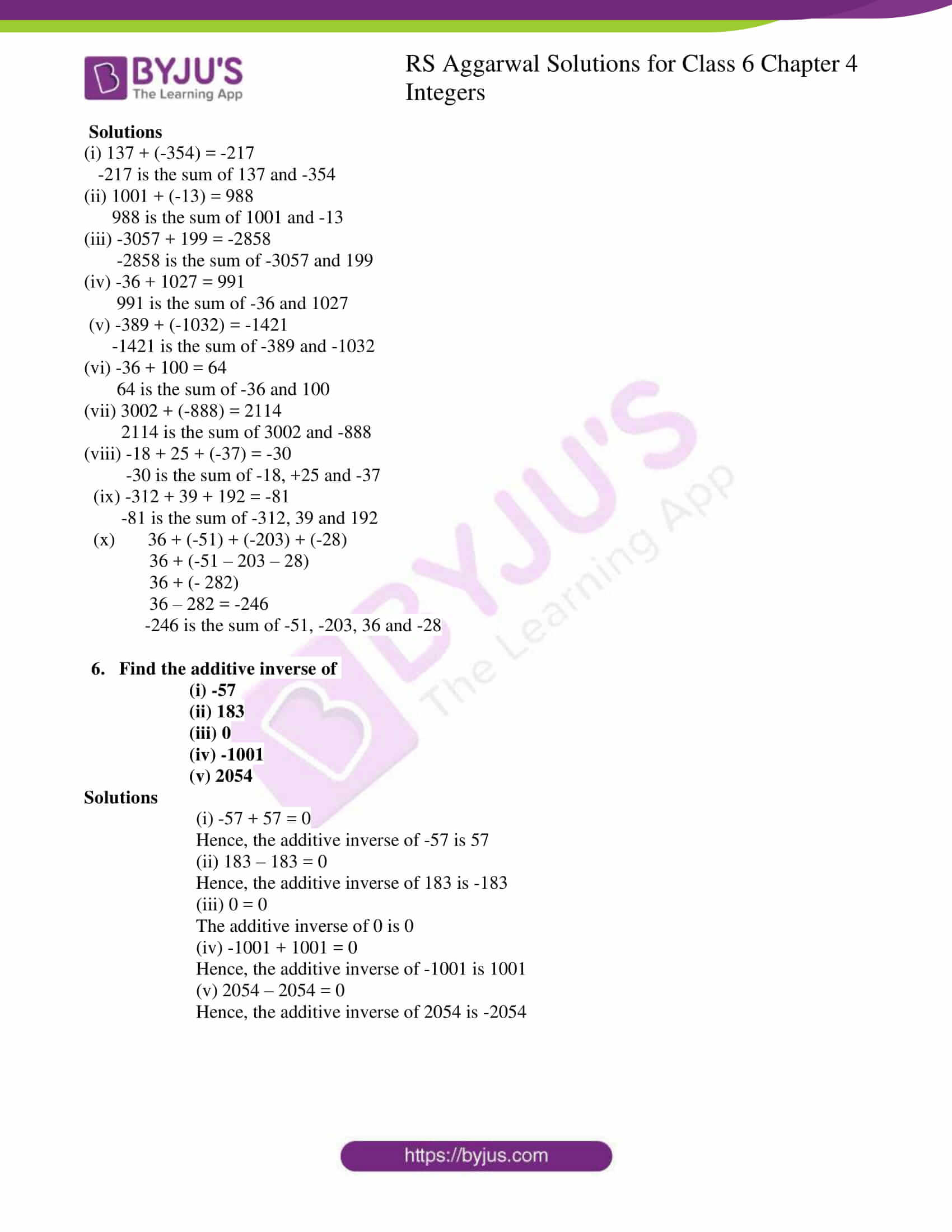## Access answers to Maths RS Aggarwal Solutions for Class 6 Chapter 4 Integers Exercise 4B

1. Use the number line and add the following integers:

(i) 9 + (-6)

(ii) (-3) + 7

(iii) 8 + (-8)

(iv) (-1) + (-3)

(v) (-4) + (-7)

(vi) (-2) + (-8)

(vii) 3 + (-2) + (-4)

(viii) (-1) + (-2) + (-3)

(ix) 5 + (-2) + (-6)

Solutions

(i) 9 + (-6) = 3

-4 -3 -2 -1 0 1 2 3 4

Hence, 3 lie on the right side of 0 on the number line

(ii) (-3) + 7 = 4

-4 -3 -2 -1 0 1 2 3 4

Hence, 4 lie on the right side of 0 on the number line

(iii) 8 + (-8) = 0

-4 -3 -2 -1 0 1 2 3 4

Hence 0 lies in the center on the number line

(iv) (-1) + (-3) = -4

-4 -3 -2 -1 0 1 2 3 4

Hence, -4 lie on right side of 0 on the number line

(v) (-4) + (-7) = -11

-11-10 -9 -8 -7 -6 -5 -4 -3 -2 -1 0 1 2 3 4

Hence, -11 lie on the right side of 0 on the number line

(vi) (-2) + (-8) = -10

-10 -9 -8 -7 -6 -5 -4 -3 -2 -1 0 1 2 3 4 5

Hence, -10 lie on the left side of 0 on the number line

(vii) 3 + (-2) + (-4) = -3

-4 -3 -2 -1 0 1 2 3 4

Hence, -3 lie on the left side of 0 on the number line

(viii) (-1) + (-2) + (-3) =-6

-7 -6 -5 -4 -3 -2 -1 0 1 2 3 4 5 6 7

Hence, -6 lie on the left side of 0 on the number line

(ix) 5 + (-2) + (-6) = -3

-5 -4 -3 -2 -1 0 1 2 3 4 5

Hence, -3 lie on the left side of 0 on the number line

2. Fill in the blanks:

(i) (-3) + (-9) =………

(ii) (-7) + (-8) = ……

(iii) (-9) + 16 = ………

(iv) (-13) + 25 = ………

(v) 8 + (-17) =………

(vi) 2 + (-12) = ………..

Solutions

(i) (-3) + (-9) = -12

(ii) (-7) + (-8) = -15

(iii) (-9) + 16 = 7

(iv) (-13) + 25 = 12

(v) 8 + (-17) = -9

(vi) 2 + (-12) = -10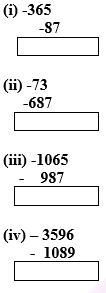Solutions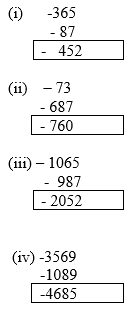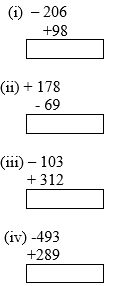Solutions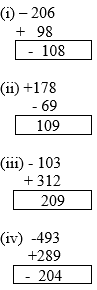5. Find the sum of

(i) 137 and – 354

(ii) 1001 and -13

(iii) -3057 and 199

(iv) -36 and 1027

(v) -389 and -1032

(vi) -36 and 100

(vii) 3002 and -888

(viii) -18, +25 and -37

(ix) -312, 39 and 192

(x) -51, -203, 36 and -28

Solutions

(i) 137 + (-354) = -217

-217 is the sum of 137 and -354

(ii) 1001 + (-13) = 988

988 is the sum of 1001 and -13

(iii) -3057 + 199 = -2858

-2858 is the sum of -3057 and 199

(iv) -36 + 1027 = 991

991 is the sum of -36 and 1027

(v) -389 + (-1032) = -1421

-1421 is the sum of -389 and -1032

(vi) -36 + 100 = 64

64 is the sum of -36 and 100

(vii) 3002 + (-888) = 2114

2114 is the sum of 3002 and -888

(viii) -18 + 25 + (-37) = -30

-30 is the sum of -18, +25 and -37

(ix) -312 + 39 + 192 = -81

-81 is the sum of -312, 39 and 192

(x) 36 + (-51) + (-203) + (-28)

36 + (-51 – 203 – 28)

36 + (- 282)

36 – 282 = -246

-246 is the sum of -51, -203, 36 and -28

6. Find the additive inverse of

(i) -57

(ii) 183

(iii) 0

(iv) -1001

(v) 2054

Solutions

(i) -57 + 57 = 0

Hence, the additive inverse of -57 is 57

(ii) 183 – 183 = 0

Hence, the additive inverse of 183 is -183

(iii) 0 = 0

The additive inverse of 0 is 0

(iv) -1001 + 1001 = 0

Hence, the additive inverse of -1001 is 1001

(v) 2054 – 2054 = 0

Hence, the additive inverse of 2054 is -2054